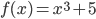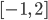### Day 36 - Increasing/Decreasing Functions - 10.06.15

Update
• Summative Exam 1 will be graded sometime today!

Bell Ringer

1. What are critical numbers?

1. numbers needed to solve problems

2. values of x that cause a derivative to be equal to zero

3. values of y that cause a derivative to be equal to zero

4. values of x that cause a derivative to be undefined

5. both b and d

2. How are relative extrema related to critical numbers?

1. There is no relationship between critical numbers and relative extrema.

2. Every relative extrema occurs at a critical number.

3. Every critical number has a relative extrema.

4. Critical numbers are only found at relative extrema.

5. none of the above

3. Find the critical number(s) of the following:1. x = 1

2. x = -1

3. x = 0

4. undefined

5. none of the above

4. Find the relative extrema for the following function:1. (1, 6)

2. (-1, 4)

3. (0, 5)

4. no relative extrema

5. none of the above

5. Find and describe all extrema for the following function:on the interval1. Absolute max: (-1, 4) | Absolute min: (2, 13) | No relative extrema

2. Absolute min: (-1, 4) | Absolute max: (2, 13) | Relative min: (0, 5)

3. Absolute min: (-1, 4) | Absolute max: (2, 13) | No relative extrema

4. No extrema

5. none of the above

Review
• Pre-calculus
• Extrema
• Minima
• Maxima
• Absolute
• Relative
• Interval Notation
• Extrema (video) (checkpoints)
• How can extrema be defined for a function?
• How can critical numbers be calculated using derivatives?
• How are critical numbers related to extrema?
• Absolute and Relative Extrema (video)
• Critical Numbers (video)

Lesson
Exit Ticket
• Posted on the board at the end of the block (link).

Homework
• N/A

#### In-Class Help Requests

Standard(s)
• APC.7
• Analyze the derivative of a function as a function in itself.
• Includes:
• comparing corresponding characteristics of the graphs of f, f', and f''
• ​defining the relationship between the increasing and decreasing behavior of f and the sign of f'
• ​translating verbal descriptions into equations involving derivatives and vice versa
• analyzing the geometric consequences of the Mean Value Theorem;
• defining the relationship between the concavity of f and the sign of f"; and ​identifying points of inflection as places where concavity changes and finding points of inflection.
• APC.8
• Apply the derivative to solve problems.
• Includes:
• ​analysis of curves and the ideas of concavity and monotonicity
• optimization involving global and local extrema;
• modeling of rates of change and related rates;
• use of implicit differentiation to find the derivative of an inverse function;
• interpretation of the derivative as a rate of change in applied contexts, including velocity, speed, and acceleration; and
• differentiation of nonlogarithmic functions, using the technique of logarithmic differentiation.*
• *AP Calculus BC will also apply the derivative to solve problems.
• Includes:
• ​analysis of planar curves given in parametric form, polar form, and vector form, including velocity and acceleration vectors;
• ​numerical solution of differential equations, using Euler’s method;
• ​l’Hopital’s Rule to test the convergence of improper integrals and series; and
• ​geometric interpretation of differential equations via slope fields and the relationship between slope fields and the solution curves for the differential equations.